# Python in Finance¶

Dr. Yves J. Hilpisch

Continuum Analytics Europe GmbH

www.continuum.io

yves@continuum.io

@dyjh

New York City – 27. August 2013

A brief bio:

• Managing Director Europe of Continuum Analytics Inc.
• Founder of Visixion GmbH – The Python Quants
• Lecturer Mathematical Finance at Saarland University
• Focus on Financial Industry and Financial Analytics
• Book (2013) "Derivatives Analytics with Python"
• Book project "Python for Finance"
• Dr.rer.pol in Mathematical Finance
• German and Martial Arts fan

## Python in Finance¶

This talk focuses on

• financial algorithm implementation
• interactive financial analytics
• prototyping-like Python usage

It does not address such important issues like

• architectural issues regarding hardware and software
• development processes, testing, documentation and production
• real world financial product modeling
• market microstructure and market conventions
• trade execution, risk management, reporting
• others like regulation

## Array-based Simulation with NumPy¶

The majority of financial algorithms, e.g. in option pricing, can be casted to an array (matrix) setting. It is therefore paramount to have available flexible and fast array manipulation capabilities.

NumPy provides the basic infrastructure for that. It provides the basic building blocks on which to build financial algorithms.

There are two major advantages:

• syntax: through vectorization/matrix notatation, NumPy code is much more compact, easier to write, to read and to maintain
• performance: NumPy is mainly implemented in C/Fortran such that operations on the NumPy level are generally much faster than pure Python

We simulate the evolution of a stock index level which we assume to follow a geometric Brownian motion

$dS_t = r S_t dt + \sigma S_t dZ_t$

with $S$ the stock price, $r$ the risk-free rate, $\sigma$ the volatility and $Z$ a Brownian motion.

An exact discretization scheme is given for $t>0$ by

$S_t = S_{t - \Delta t} \exp \left((r - 0.5 \sigma^2) \Delta t + \sigma \sqrt{\Delta t} z_t \right)$

with $z$ being a standard normally distributed random variable.

### Simulation with Pure Python¶

First, we import needed functions and define some global variables.

In :
#
# Simulating Geometric Brownian Motion with Python
#
from time import time
from math import exp, sqrt, log
from random import gauss

# Parameters
S0 = 100; r = 0.05; sigma = 0.2
T = 1.0; M = 50; dt = T / M


Then we define a function which returns us I simulated index level paths.

In :
# Simulating I paths with M time steps
def genS_py(I):
''' I: number of paths '''
S = []
for i in range(I):
path = []
for t in range(M + 1):
if t == 0:
path.append(S0)
else:
z = gauss(0.0, 1.0)
St = path[t - 1] * exp((r - 0.5 * sigma ** 2) * dt
+ sigma * sqrt(dt) * z)
path.append(St)
S.append(path)
return S


Let's see how long the simulation takes.

In :
I = 100000
%time S = genS_py(I)

CPU times: user 18.8 s, sys: 152 ms, total: 19 s
Wall time: 19.4 s


In :
plot(S)
grid(True)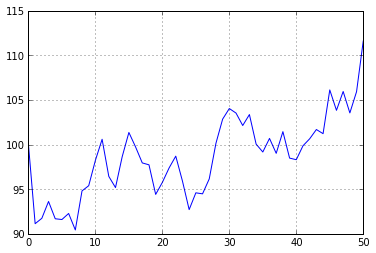### Simulation with NumPy¶

We use the log version of the discretization scheme to fully apply NumPy capabilities.

In :
from numpy import *
def genS_np(I):
''' I: number of paths '''
S = S0 * exp(cumsum((r - 0.5 * sigma ** 2) * dt
+ sigma * sqrt(dt) * standard_normal((M + 1, I)), axis=0))
S = S0
return S

In :
%time S = genS_np(I)

CPU times: user 884 ms, sys: 184 ms, total: 1.07 s
Wall time: 1.1 s



This leads to a considerable speed-up and to a quite compact syntax – the algorithm is "fully NumPy".

## Binomial Option Pricing with NumPy¶

### Binomial Option Pricing with Python Loops¶

First, the parameters for pricing an option on a stock index level.

In :
from math import exp, sqrt

# Option Parameters
S0 = 105.0  # initial index level
K = 100.0  # strike price
T = 1.  # call option maturity
r = 0.05  # constant short rate
vola = 0.25  # constant volatility factor of diffusion

# Time Parameters
M = 1000  # time steps
dt = T / M  # length of time interval
df = exp(-r * dt)  # discount factor per time interval

# Binomial Parameters
u = exp(vola * sqrt(dt))  # up-movement
d = 1 / u  # down-movement
q = (exp(r * dt) - d) / (u - d)  # martingale probability


The loop-heavy implementation which is already based on NumPy arrays.

In :
import numpy as np
def oVal_py():
# Array Initialization for Index Levels
S = np.zeros((M + 1, M + 1), dtype=np.float64)  # index level array
S[0, 0] = S0
z = 0
for j in range(1, M + 1, 1):
z += 1
for i in range(z + 1):
S[i, j] = S[0, 0] * (u ** j) * (d ** (i * 2))
# Array Initialization for Inner Values
iv = np.zeros((M + 1, M + 1), dtype=np.float64)  # inner value array
z = 0
for j in range(0, M + 1, 1):
for i in range(z + 1):
iv[i, j] = max(S[i, j] - K, 0)
z += 1
# Valuation by Risk-Neutral Discounting
pv = np.zeros((M + 1, M + 1), dtype=np.float64)  # present value array
pv[:, M] = iv[:, M]  # initialize last time point
z = M + 1
for j in range(M - 1, -1, -1):
z -= 1
for i in range(z):
pv[i, j] = (q * pv[i, j + 1] + (1 - q) * pv[i + 1, j + 1]) * df
return pv[0, 0]


The execution takes quite a bit of time.

In :
%timeit C = oVal_py()

1 loops, best of 3: 6.31 s per loop



### Performance Improvements with Numba¶

Numba is an open-source, NumPy-aware optimizing compiler for Python sponsored by Continuum Analytics, Inc. It uses the remarkable LLVM compiler infrastructure to compile Python byte-code to machine code especially for use in the NumPy run-time and SciPy modules.

From Numba we can expect some nice performance improvements ...

In :
from numba import *
oVal_nb = autojit(oVal_py)

In :
%timeit C = oVal_nb()

1 loops, best of 3: 282 ms per loop



... with only one two lines of additional code.

### Binomial Option Pricing with Numpy¶

The NumPy version avoids all but one loop on the Python level.

In :
def oVal_np():
# Array Initialization for Index Levels
mu = arange(M + 1)
mu = resize(mu, (M + 1, M + 1))
md = transpose(mu)
mu = u ** (mu - md)
md = d ** md
S = S0 * mu * md

# Valuation Algorithm
V = maximum(S - K, 0)  # inner values for European call option
Qu = zeros((M + 1, M + 1), dtype=np.float64)
Qu[:, :] = q  # upwards martingale probabilities
Qd = 1 - Qu  # downwards martingale probabilities
z = 0
for t in range(M - 1, -1, -1):  # backwards iteration
V[0:M - z, t] = (Qu[0:M - z, t] * V[0:M - z, t + 1]
+ Qd[0:M - z, t] * V[1:M - z + 1, t + 1]) * df
z += 1
return V[0, 0]


The NumPy version approximately as fast as the Numba version.

In :
%timeit C = oVal_np()

1 loops, best of 3: 349 ms per loop



## Parallel Execution of Option Pricing Code¶

This example illustrates how to implement a parallel valuation of American options by Monte Carlo simulation. The algorithm used is the Least-Squares Monte Carlo algorithm as proposed in Longstaff-Schwartz (2001): "Valuing American Options by Simulation: A Simple Least-Squares Approach." Review of Financial Studies, Vol. 14, 113-147. We observe speed-ups that are almost linear in the number of cores.

### The LSM Algorithm¶

The following is an implementation of the Least-Squares Monte Carlo algorithm (LSM) of Longstaff-Schwartz (2001).

In :
def optionValue(S0, vol, T, K=40, M=50, I=4096, r=0.06):
import numpy as np
np.random.seed(150000)  # fix the seed for every valuation
dt = T / M  # time interval
df = np.exp(-r * dt)  # discount factor per time time interval
# Simulation of Index Levels
S = np.zeros((M + 1, I), dtype=np.float64)  # stock price matrix
S[0, :] = S0  # intial values for stock price
for t in range(1, M + 1):
ran = np.random.standard_normal(I / 2)
ran = np.concatenate((ran, -ran))  # antithetic variates
ran = ran - np.mean(ran)  # correct first moment
ran = ran / np.std(ran)  # correct second moment
S[t, :] = S[t - 1, :] * np.exp((r - vol ** 2 / 2) * dt
+ vol * ran * np.sqrt(dt))
h = np.maximum(K - S, 0)  # inner values for put option
V = np.zeros_like(h)  # value matrix
V[-1] = h[-1]
# Valuation by LSM
for t in range(M - 1, 0, -1):
rg = np.polyfit(S[t, :], V[t + 1, :] * df, 5)  # regression
C = np.polyval(rg, S[t, :])  # evaluation of regression
V[t, :] = np.where(h[t, :] > C, h[t, :],
V[t + 1, :] * df)  # exercise decision/optimization
V0 = np.sum(V[1, :] * df) / I  # LSM estimator
print "S0 %4.1f|vol %4.2f|T %2.1f| Option Value %8.3f" % (S0, vol, T, V0)
return V0


### The Sequential Calculation¶

We want to replicate the whole table 1 of the seminal paper.

In :
def seqValue():
optionValues = []
for S0 in (36., 38., 40., 42., 44.):  # initial stock price values
for vol in (0.2, 0.4):  # volatility values
for T in (1.0, 2.0):  # times-to-maturity
optionValues.append(optionValue(S0, vol, T))
return optionValues


Now, we measure the time for the 20 different American put options of that table 1 with sequential execution.

In :
from time import time
t0 = time()
optionValues = seqValue()  # calculate all values
t1 = time(); d1 = t1 - t0
print "Duration in Seconds %6.3f" % d1

S0 36.0|vol 0.20|T 1.0| Option Value    4.500
S0 36.0|vol 0.20|T 2.0| Option Value    4.854
S0 36.0|vol 0.40|T 1.0| Option Value    7.112


### The Parallel Calculation¶

First, start a local cluster, if you have multiple cores in your machine.

In :
# in the shell ...
####
# ipcluster start -n 2
####
# or maybe more cores


Enable parallel computing capabilities.

In :
from IPython.parallel import Client
cluster_profile = "default"
# the profile is set to the default one
# see IPython docs for further details
c = Client(profile=cluster_profile)


Again, a loop for the 20 options of table 1. This time asynchronously distributed to the 2 cores.

In :
def parValue():
optionValues = []
for S in (36., 38., 40., 42., 44.):
for vol in (0.2, 0.4):
for T in (1.0, 2.0):
value = view.apply_async(optionValue, S, vol, T)
# asynchronously calculate the option values
optionValues.append(value)
return optionValues


Now, we measure the time needed with parallel execution.

In :
def execution():
optionValues = parValue()  # calculate all values
print "Submitted tasks %d" % len(optionValues)
c.wait(optionValues)
# wait for all tasks to be finished
return optionValues
t0 = time()
optionValues = execution()
t1 = time(); d2 = t1 - t0
print "Duration in Seconds %6.3f" % d2

Submitted tasks 20
Duration in Seconds  2.712


In :
d1 / d2  # speed-up of parallel execution

Out:
1.6769731386025957


You can inspect the metadata and access single data fields from the results dictionary.

In :
optionValues.metadata

Out:
{'after': [],
'completed': datetime.datetime(2013, 8, 27, 18, 2, 17, 793919),
'data': {},
'engine_id': 0,
'engine_uuid': u'597b14f1-ecfb-4a50-a35d-aa44897997b9',
'follow': [],
'msg_id': u'089767bb-c1f6-4a22-9daa-8247ef700f74',
'outputs': [],
'pyerr': None,
'pyin': None,
'pyout': None,
'received': datetime.datetime(2013, 8, 27, 18, 2, 17, 798400),
'started': datetime.datetime(2013, 8, 27, 18, 2, 17, 511909),
'status': u'ok',
'stderr': '',
'stdout': u'S0 36.0|vol 0.20|T 1.0| Option Value    4.500\n',
'submitted': datetime.datetime(2013, 8, 27, 18, 2, 17, 506365)}


The whole output of the 20 valuations.

In :
for result in optionValues:

S0 36.0|vol 0.20|T 1.0| Option Value    4.500
S0 36.0|vol 0.20|T 2.0| Option Value    4.854
S0 36.0|vol 0.40|T 1.0| Option Value    7.112
S0 36.0|vol 0.40|T 2.0| Option Value    8.472
S0 38.0|vol 0.20|T 1.0| Option Value    3.262
S0 38.0|vol 0.20|T 2.0| Option Value    3.723
S0 38.0|vol 0.40|T 1.0| Option Value    6.112
S0 38.0|vol 0.40|T 2.0| Option Value    7.682
S0 40.0|vol 0.20|T 1.0| Option Value    2.305
S0 40.0|vol 0.20|T 2.0| Option Value    2.861
S0 40.0|vol 0.40|T 1.0| Option Value    5.258
S0 40.0|vol 0.40|T 2.0| Option Value    6.899
S0 42.0|vol 0.20|T 1.0| Option Value    1.569
S0 42.0|vol 0.20|T 2.0| Option Value    2.164
S0 42.0|vol 0.40|T 1.0| Option Value    4.544
S0 42.0|vol 0.40|T 2.0| Option Value    6.171
S0 44.0|vol 0.20|T 1.0| Option Value    1.020
S0 44.0|vol 0.20|T 2.0| Option Value    1.602
S0 44.0|vol 0.40|T 1.0| Option Value    3.912
S0 44.0|vol 0.40|T 2.0| Option Value    5.591



## Calibration of Volatility Option Pricing Model¶

The following example is taken from Eurex's VSTOXX Advanced Services intiative which uses Python to illustrate volatility concepts and volatilty product features, e.g. of VSTOXX Futures and VSTOXX options. The complete tutorials and Python scripts are available under

### The Pricing Function for European Volatility Options¶

In the model of Andreas Gruenbichler and Francis Longstaff (1996): "Valuing Futures and Options on Volatility." Journal of Banking and Finance, Vol. 20, 985 – 1001, (implied) volatility is assumed to follow a square-root diffusion process

$dV_t = \kappa (\theta - V_t) dt + \sigma \sqrt{V_t} dZ_t$

where $Z$ is a Brownian motion. GL96 derive a semi-analytical pricing formula for European call if volatility follows this stochastic differential equation. The following two Python functions implement their formula.

First, a helper function for the Chi-Squared Density.

In :
def cx(K, gamma, nu, lambda_V):
'''Complementary Distribution Function of Non-Central Chi-Squared Density
K: strike price
gamma: as defined in the GL96 model
nu: degrees of freedom
lambda_V: non-centrality parameter '''
from scipy.stats import ncx2
return 1 - ncx2.cdf(gamma * K, nu, lambda_V)


Now the option pricing function for European calls on volatility.

In :
def eurCallGL96(V0, kappa_V, theta_V, sigma_V, zeta_V, T, r, K):
'''Calcuation of European Call Option Price in GL96 Model
V0: current volatility level
kappa_V: mean-reversion factor
theta_V: long-run mean of volatility
sigma_V: volatility of volatility
zeta_V: volatility risk premium
T: time-to-maturity
r: risk-free short rate
K: strike price of the option '''
from math import exp
D = exp(-r * T)  # discount factor
# variables
alpha = kappa_V * theta_V
beta = kappa_V + zeta_V
gamma = 4 * beta / (sigma_V ** 2 * (1 - exp(-beta * T)))
nu = 4 * alpha / sigma_V ** 2
lambda_V = gamma * exp(-beta * T) * V0
# formula for European call price
C = D * exp(-beta * T) * V0 * cx(K, gamma, nu + 4, lambda_V) \
+ D * (alpha / beta) * (1 - exp(-beta * T)) \
* cx(K, gamma, nu + 2, lambda_V) \
- D * K * cx(K, gamma, nu, lambda_V)
return C


A simple pricing example.

In :
# Model Parameters
V0 = 21.39          # initial level of volatility index
kappa_V = 0.1       # speed of mean reversion
theta_V = 20.0      # long-term index level
sigma_V = 2.0       # volatility of volatility
zeta_V = 0.0        # factor of the expected volatility risk premium
r = 0.02            # risk-free interest rate

# Option Parameters
K = 22.0            # strike
T = 1.0             # time horizon

C = eurCallGL96(V0, kappa_V, theta_V, sigma_V, zeta_V, T, r, K)
print "Value of European Call according to GL96:   %10.4f" % C

Value of European Call according to GL96:       3.0969



### Calibration of the Model to Market Quotes¶

We first need to import some market data to calibrate the model to.

In :
import pandas as pd
h5 = pd.HDFStore('VSTOXX_Opt_Fut.h5', 'r')
vso_mar = h5['vso_mar']
h5.close()


Some selected data from the DataFrame object.

In :
vso_mar[['Strike Price', 'Last Price', 'Date', 'Time']].ix[10:20]

Out:
Strike Price Last Price Date Time
10 35.0 0.75 12/28/2012 18:33:48
11 32.5 0.90 12/28/2012 18:33:48
12 30.0 1.10 12/28/2012 18:33:48
13 29.0 1.20 12/28/2012 18:33:48
14 28.0 1.35 12/28/2012 18:33:48
15 27.0 1.45 12/28/2012 18:33:48
16 26.0 1.65 12/28/2012 18:33:48
17 25.0 1.85 12/28/2012 18:33:48
18 24.0 2.05 12/28/2012 18:33:48
19 23.0 2.35 12/28/2012 18:33:48
20 22.0 2.70 12/28/2012 18:33:48

We take a sub-set of the option data for the calibration and define some fixed parameters.

In :
kL = np.array(vso_mar['Strike Price'][12:25])
vL = np.array(vso_mar['Last Price'][12:25])

In :
# Fixed Parameters
days = 3 + 31 + 28 + 15  # time-to-maturity in days
T = days / 365.  # time-to-maturity in years
r = 0.01  # risk-less short rate
V0 = 21.3534  # starting level of VSTOXX (28.12.2012)
zeta_V = 0.  # volatility risk premium factor


Now, we define a function which gives us back an array of the whole set of model option prices.

In :
def valFunc(p0):
'''Valuation Function for Array of Strike Prices
p0: set of parameters for calibration '''
kappa_V, theta_V, sigma_V = p0
GL96val = []
for K in kL:
GL96val.append(eurCallGL96(V0, kappa_V, theta_V,
sigma_V, zeta_V, T, r, K))
return np.array(GL96val)


Next, the error function which is to be minimized.

In :
def errFunc(p0):
'''Error Function for GL96 Calibration
p0: set of parameters for calibration '''
GL96val = valFunc(p0)
kappa_V, theta_V, sigma_V = p0
pen = 0.
if 2 * kappa_V * theta_V < sigma_V ** 2:
pen = 1000.0  # penalty if Feller condition is not met
if kappa_V < 0 or theta_V < 0 or sigma_V < 0:
pen = 1000.0  # penalty for negative parameters
MSE = sum((GL96val - vL) ** 2) / len(vL) + pen
# print p0.round(3), "%16.4f" % MSE
return MSE


The calibration strategy rests on both global and local minimization of the error function. The result of the former is used as input for the latter.

In :
import scipy.optimize as sop

In :
%time p0 = sop.brute(errFunc, ((1.0, 15.1, 2.0), (10., 40.1, 2.5), (2.0, 18.1, 4.0)), finish=None)

CPU times: user 4.82 s, sys: 0 ns, total: 4.82 s
Wall time: 4.85 s


In :
errFunc(p0).round(5)

Out:
0.02588


Take p0 for the the local minimization.

In :
%time opt = sop.fmin(errFunc, p0, xtol=0.000001, ftol=0.000001, maxiter=1000, maxfun=1500)

Optimization terminated successfully.
Current function value: 0.012411
Iterations: 337
Function evaluations: 589
CPU times: user 5.53 s, sys: 0 ns, total: 5.53 s
Wall time: 5.59 s



We want to inspect the quality of the calibration graphically.

In :
def genPlot(opt):
''' Function to compare model values with market quotes.
opt: (optimal) input parameter set. '''
GL96val = valFunc(opt)
figure()
subplot(211)
plot(kL, vL, label='Market Quotes')
plot(kL, GL96val, 'ro', label='Model Prices')
xlabel('Strike Price')
ylabel('Option Value')
grid(True)
legend()
axis([min(kL) - 0.5, max(kL) + 0.5, 0.0, max(vL) * 1.1])
subplot(212)
wi = 0.3
bar(kL - wi / 2, GL96val - vL, width=wi)
grid(True)
ylabel('Difference')
axis([min(kL) - 0.5, max(kL) + 0.5,
min(GL96val - vL) * 1.1, max(GL96val - vL) * 1.1])


The calibration seems not too bad.

In :
genPlot(opt)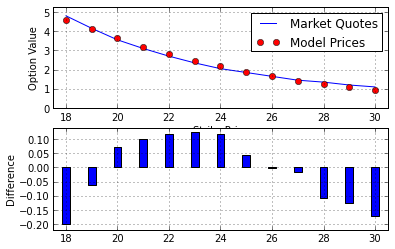## Analyzing Time Series Data¶

### Historical Correlation between EURO STOXX 50 and VSTOXX¶

It is a stylized fact that stock indexes and related volatility indexes are highly negatively correlated. The following example analyzes this based on the EURO STOXX 50 stock index and the VSTOXX volatility index using Ordinary Least-Squares regession (OLS).

First we collect historical data for both the EURO STOXX 50 stock and the VSTOXX volatility index.

In :
import pandas as pd
from urllib import urlretrieve

In :
es_url = 'http://www.stoxx.com/download/historical_values/hbrbcpe.txt'
urlretrieve(es_url, 'es.txt')
urlretrieve(vs_url, 'vs.txt')

Out:
('vs.txt', <httplib.HTTPMessage instance at 0x7278200>)


The EURO STOXX 50 data is not yet in the right format. Some house cleaning is necessary.

In :
lines = open('es.txt').readlines()
new_file = open('es50.txt', 'w')
new_file.writelines('date' + lines[:-1].replace(' ', '') + ';DEL' + lines[-1])
new_file.writelines(lines[4:-1])
print list(open('es50.txt'))[:5]

['date;SX5P;SX5E;SXXP;SXXE;SXXF;SXXA;DK5F;DKXF;DEL\n', '31.12.1986;775.00 ;  900.82 ;   82.76 ;   98.58 ;   98.06 ;   69.06 ;  645.26  ;  65.56\n', '01.01.1987;775.00 ;  900.82 ;   82.76 ;   98.58 ;   98.06 ;   69.06 ;  645.26  ;  65.56\n', '02.01.1987;770.89 ;  891.78 ;   82.57 ;   97.80 ;   97.43 ;   69.37 ;  647.62  ;  65.81\n', '05.01.1987;771.89 ;  898.33 ;   82.82 ;   98.60 ;   98.19 ;   69.16 ;  649.94  ;  65.82\n']



Now, the data can be safely read into a DataFrame object.

In :
es = pd.read_csv('es50.txt', index_col=0, parse_dates=True, sep=';', dayfirst=True)

In :
del es['DEL']  # delete the helper column
es

Out:
<class 'pandas.core.frame.DataFrame'>
DatetimeIndex: 6862 entries, 1986-12-31 00:00:00 to 2013-08-08 00:00:00
Data columns (total 8 columns):
SX5P    6862  non-null values
SX5E    6862  non-null values
SXXP    6862  non-null values
SXXE    6861  non-null values
SXXF    6861  non-null values
SXXA    6861  non-null values
DK5F    6861  non-null values
DKXF    6861  non-null values
dtypes: float64(8)


The VSTOXX data can be read without touching the raw data.

In :
vs = pd.read_csv('vs.txt', index_col=0, header=2, parse_dates=True, sep=',', dayfirst=True)

# you can alternatively read from the Web source without saving the csv file to disk:
# vs = pd.read_csv(vs_url, index_col=0, header=2, parse_dates=True, sep=',', dayfirst=True)


We now merge the data for further analysis.

In :
from datetime import datetime
data1 = pd.DataFrame({'EUROSTOXX' : es['SX5E'][es.index > datetime(1999, 12, 31)]})
data2 = pd.DataFrame({'VSTOXX' : vs['V2TX'][vs.index > datetime(1999, 12, 31)]})
data = data1.join(data2)
data

Out:
<class 'pandas.core.frame.DataFrame'>
DatetimeIndex: 3487 entries, 2000-01-03 00:00:00 to 2013-08-08 00:00:00
Data columns (total 2 columns):
EUROSTOXX    3487  non-null values
VSTOXX       3466  non-null values
dtypes: float64(2)


Let's inspect the two time series.

In :
data.head()

Out:
EUROSTOXX VSTOXX
date
2000-01-03 4849.22 30.98
2000-01-04 4657.83 33.22
2000-01-05 4541.75 32.59
2000-01-06 4500.69 31.18
2000-01-07 4648.27 27.44

A picture can tell the complete story.

In :
data.plot(subplots=True, grid=True, style='b')

Out:
array([<matplotlib.axes.AxesSubplot object at 0x4f30990>,
<matplotlib.axes.AxesSubplot object at 0x4f0dc10>], dtype=object)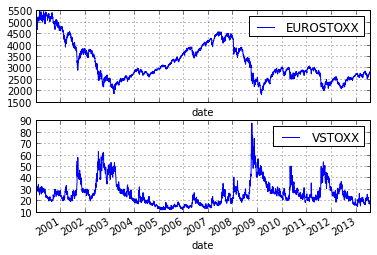We now generate log returns for both time series.

In :
rets = np.log(data / data.shift(1))

Out:
EUROSTOXX VSTOXX
date
2000-01-03 NaN NaN
2000-01-04 -0.040268 0.069810
2000-01-05 -0.025237 -0.019147
2000-01-06 -0.009082 -0.044229
2000-01-07 0.032264 -0.127775

To this new data set, also stored in a DataFrame object, we apply OLS.

In :
xdat = pd.rolling_mean(rets['EUROSTOXX'], window=1).shift(0)
ydat = pd.rolling_mean(rets['VSTOXX'], window=1).shift(0)
model = pd.ols(y=ydat, x=xdat)
model

Out:

-------------------------Summary of Regression Analysis-------------------------

Formula: Y ~ <x> + <intercept>

Number of Observations:         3444
Number of Degrees of Freedom:   2

R-squared:         0.5669

Rmse:              0.0371

F-stat (1, 3442):  4505.5960, p-value:     0.0000

Degrees of Freedom: model 1, resid 3442

-----------------------Summary of Estimated Coefficients------------------------
Variable       Coef    Std Err     t-stat    p-value    CI 2.5%   CI 97.5%
--------------------------------------------------------------------------------
x    -2.6942     0.0401     -67.12     0.0000    -2.7729    -2.6155
intercept    -0.0007     0.0006      -1.16     0.2459    -0.0020     0.0005
---------------------------------End of Summary---------------------------------



Some selected results and again a pricture to illustrate the stylized fact in graphic fashion – the results obviously support the stylized fact.

In :
print "beta %8.4f" % model.beta
print "intc %8.4f" % model.beta
print "r**2 %8.4f" % model.r2

beta  -2.6942
intc  -0.0007
r**2   0.5669



Again, we want to see how our results look graphically.

In :
plot(xdat, ydat, 'r.')
ax = axis()  # grab axis values
x = linspace(ax, ax + 0.01)
plot(x, model.beta + model.beta * x, 'b', lw=2)
grid(True)
axis('tight')

Out:
(-0.10000000000000001, 0.16, -0.43180704799170044, 0.43706494329021089)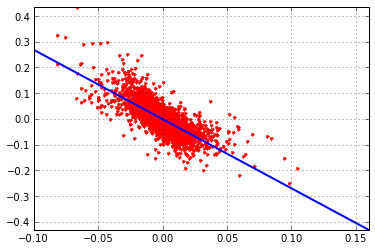### Analyzing High Frequency Data¶

Using pandas, the code that follows reads intraday, high-frequency data from a Web source, plots it and resamples it.

In :
date = datetime.now()
url = 'http://hopey.netfonds.no/posdump.php?date=%d0%d%d&paper=AAPL.O&csv_format=csv' \
% (date.year, date.month, date.day - 1)
# you may have to adjust the date since only recent dates are available

In :
AAPL

Out:
<class 'pandas.core.frame.DataFrame'>
DatetimeIndex: 3681 entries, 2013-08-26 10:00:02 to 2013-08-26 22:18:35
Data columns (total 6 columns):
bid                  3681  non-null values
bid_depth            3681  non-null values
bid_depth_total      3681  non-null values
offer                3681  non-null values
offer_depth          3681  non-null values
offer_depth_total    3681  non-null values
dtypes: float64(2), int64(4)


The intraday evolution of the Apple stock price.

In :
AAPL['bid'].plot()

Out:
<matplotlib.axes.AxesSubplot at 0x4f39fd0>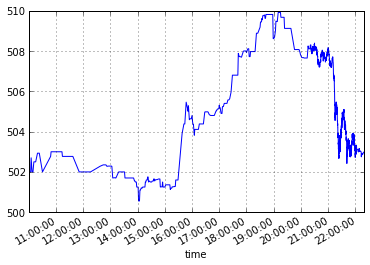A resampling of the data is easily accomplished with pandas.

In :
# this resamples the record frequency to 5 minutes, using mean as aggregation rule
AAPL_5min = AAPL.resample(rule='5min', how='mean')

Out:
bid bid_depth bid_depth_total offer offer_depth offer_depth_total
time
2013-08-26 10:00:00 502.000000 400 400 503.700000 175.000000 175.000000
2013-08-26 10:05:00 502.233333 400 400 503.206667 233.333333 233.333333
2013-08-26 10:10:00 502.500000 200 200 503.620000 500.000000 500.000000
2013-08-26 10:15:00 502.715000 200 200 503.620000 200.000000 200.000000
2013-08-26 10:20:00 502.930000 200 200 503.510000 100.000000 100.000000

Let's have a look at the new data set.

In :
AAPL_5min['bid'].plot()

Out:
<matplotlib.axes.AxesSubplot at 0x384b450>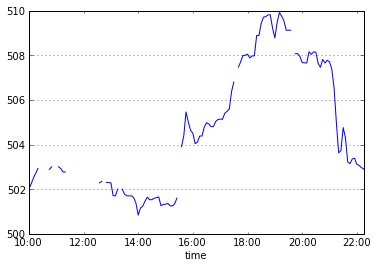Obvioulsly, there hasn't been trading data for every interval.

pandas has a number of approaches to fill in missing data.

In :
AAPL_5min['bid'].fillna(method='ffill').plot()

Out:
<matplotlib.axes.AxesSubplot at 0x3a48250>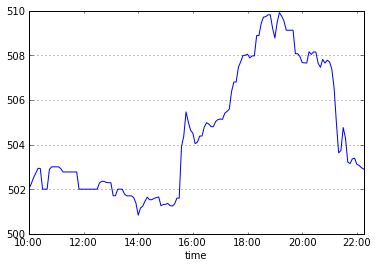## Why Python in Finance?¶

10 years ago, Python was considered exotic in Finance – at best. Today, Python has become a major force in Finance due to a number of characteristics:

• multi-purpose: prototyping, development, production, sytems administration – Python is one for all
• libraries: there is a library for almost any task or problem you face
• efficiency: Python speeds up all IT development tasks financial institutions typically face and reduces maintenance costs
• performance: Python has evolved from a scripting language to a 'meta' language with bridges to all high performance environments (e.g. LLVM, multi-core CPUs, GPUs, clusters)
• interoperalbility: Python seamlessly integrates with almost any other language and technology
• interactivity: Python allows domain experts to get closer to their business and financial data pools and to do real-time analytics## Continuum Analytics Europe GmbH – Python Data Exploration & Visualization¶

Continuum Analytics, Inc. – the company Web site

www.continuum.io

Dr. Yves J. Hilpisch – my personal Web site

www.hilpisch.com

Derivatives Analytics with Python – my new book

Read an Excerpt and Order the Book

yves@continuum.io

@dyjh

## Continuum Analytics, Inc.¶

Continuum Analytics is about Python Data Exploration & Visualization.

The team comprises, among others:

• Travis Oliphant (CEO)
• Peter Wang (President)
• Francesc Alted (Software Architect)
• Marc Wiebe (Software Architect)

Continuum provides the Python community with a number of open source libraries and free solutions to build Python-based data analytics infrastructures:

• Anaconda – Python distribution
• Wakari – Web-based, scalable, collaborative data analytics with e.g. IPython Notebook
• Numba – just-in-time compiler for CPU code
• Blaze – next generation arrays ("Data Web")
• Binstar – solving the last mile problem of Python packaging

In June 2013, Continuum Analytics opened its first office and a separate business untit in Europe. We are actively recruiting and are looking for Python enthusiasts with diverse backgrounds.